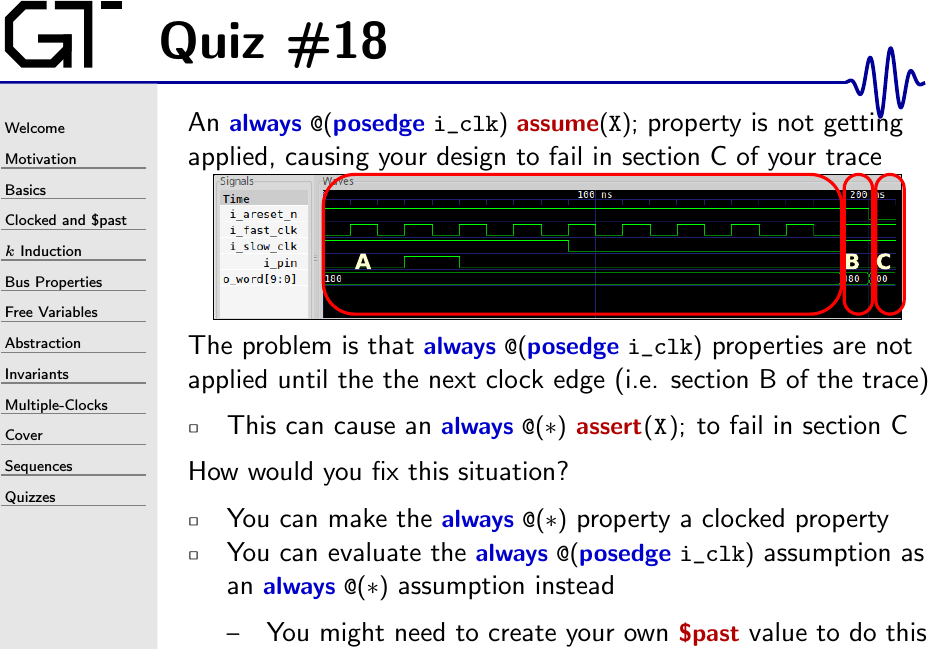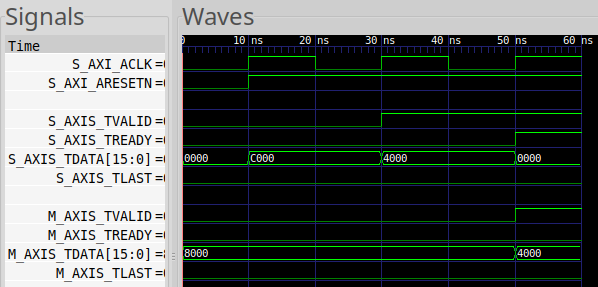Perhaps an example might help explain this one.

Suppose you have the following AXI-stream example where you want to forward an incoming AXI-stream (slave) to an outgoing AXI-stream master.

To make this work, you’d use the basic AXI stream properties. For now, let’s just focus on the input assumptions–although you’d need another set of similar assertions on the master port.

This should be fairly straightforward–you are just forwarding the stream along. Because there’s no skid buffer in this example, the design would be limited to 50% throughput.

This is all pretty basic so far.

Let’s make this a bit more challenging: We’ll insist that the example be “multiclock”–separating the clock itself from the formal timestep. This means that we’ll need some stability assumptions as well–specifically, assuming that input values will only change on a clock tick.

Still, this remains pretty basic.

One of the consequences of our implementation above, and specifically of not using a skid buffer, is that we forward the data to the downstream master and set `S_AXIS_TREADY` while the slave is stalled. That means that, on the same clock the data is accepted, it should already be valid on `M_AXIS_TDATA`.

Shall we verify this?

Unfortunately, this assertion will fail with the trace shown below.Wait, what? Why??

It will fail because the assumption that keeps `S_AXIS_TDATA` from changing if the slave is stalled doesn’t get applied until the next clock cycle. That’s what the picture in the slide above is there to help describe. In this case, `S_AXIS_TDATA` will follow its stability assumption in regions A and B, but not yet in region C since there’s been no clock tick in region C yet. The clock tick won’t take place for another cycle, pushing region C into region B.

There are two potential solutions to this problem.

1. We could take the failing assertion and move it from `always @(*)` to a test guarded by `always @(posedge S_AXI_ACLK)`. This may be the simplest fix. This fix would work for this problem.

The problem is that I tend to like the `always @(*)` criteria. This has forced me into situations where some other assertion elsewhere in the design would take more work to adjust and so I’ve then been constrained to do this the other way.

2. The other way is to implement your own `\$past()` value. Once you see it, it’s easy to do–just annoying. In this case, we might do the following:

Once you do this, you can then move the stability assertion to a combinatorial block.

This would also solve your problem.

I should point out, however, that there is a computational penalty to using an `always @(*)` formal property in a simulation environment. The problem is that the property will be re-evaluated every time something it depends upon changes. (This is normally what you would want …) A property built upon `always @(posedge clk)`, on the other hand, will only be evaluated on the clock edge, simplifying the amount of processing required by the simulator. If your goal, therefore, is to use formal properties with both simulations and formal methods, it might make more sense to instead convert all of your checks to `@(posedge i_clk)`.

I’ll let you be the judge.